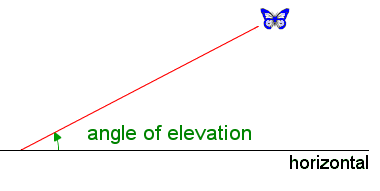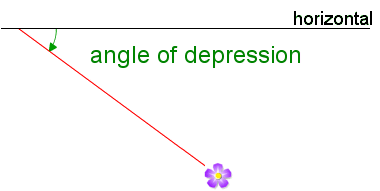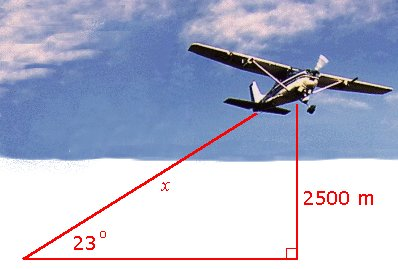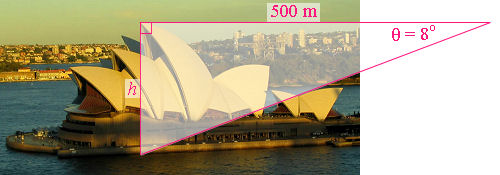Search IntMath
Close

450+ Math Lessons written by Math Professors and Teachers

5 Million+ Students Helped Each Year

1200+ Articles Written by Math Educators and Enthusiasts

Simplifying and Teaching Math for Over 23 Years

# 4. The Right Triangle and Applications

Many problems involve right triangles. We often need to use the trigonometric ratios to solve such problems.

### Example 1 - Finding the Height

Find h for the given triangle.

15.70o

Triangle with unknown height h.

sin 15.70^"o" = h/2.500

h = 2.500\ sin 15.70^"o"

= 0.6765\ "km"

### Example 2 - Solving Triangles

Solve the triangle ABC, given that A = 35° and c = 15.67.

To "solve" a triangle means to find the unknown sides and angles.

We have, for the values given:

In this example, we need to find side lengths a and b and angle B. Note C = 90o.

sin 35^"o" = a/c = a/15.67

So a = 15.67 sin 35o = 8.99

cos 35^"o"=b/c=b/15.67

So b = 15.67 cos 35o = 12.84

Angle B = 90o − 35o = 55o.

So a = 8.99, b = 12.84 and B = 55o. We have found all the unknowns.

## Angles of Elevation and Depression

In surveying, the angle of elevation is the angle from the horizontal looking up to some object:The angle of depression is the angle from the horizontal looking down to some object:### Example 3

The angle of elevation of an aeroplane is 23°. If the aeroplane's altitude is 2500\ "m", how far away is it?Let the distance be x. Then sin 23^"o"=2500/x

x=2500/(sin 23^"o")=6400\ "m"

### Example 4

You can walk across the Sydney Harbour Bridge and take a photo of the Opera House from about the same height as top of the highest sail.This photo was taken from a point about 500\ "m" horizontally from the Opera House and we observe the waterline below the highest sail as having an angle of depression of 8°. How high above sea level is the highest sail of the Opera House?This is a simple tan ratio problem.

tan 8^"o" = h/500

So

h= 500\ tan 8° = 70.27\ "m".

So the height of the tallest point is around 70\ "m".

[The actual height is 67.4\ "m".]

## Problem SolverThis tool combines the power of mathematical computation engine that excels at solving mathematical formulas with the power of GPT large language models to parse and generate natural language. This creates math problem solver thats more accurate than ChatGPT, more flexible than a calculator, and faster answers than a human tutor. Learn More.